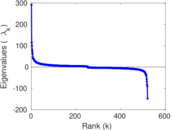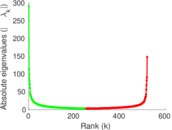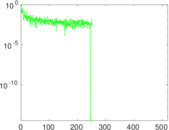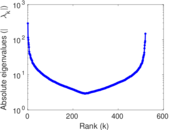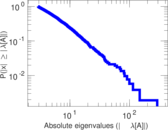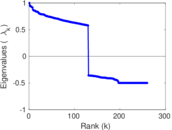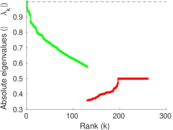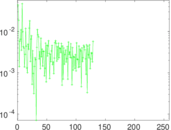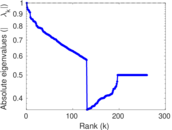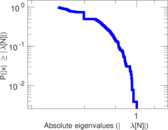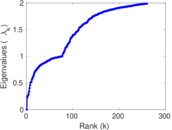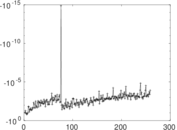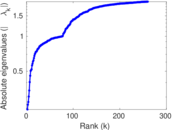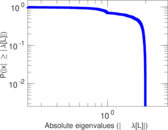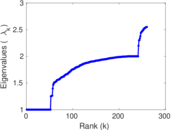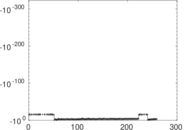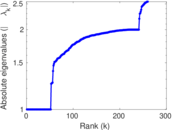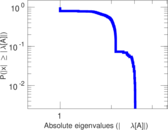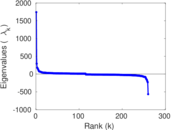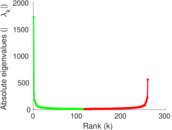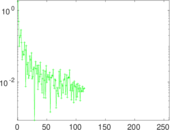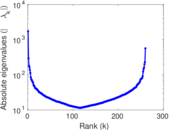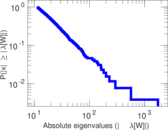# Bible

This undirected network contains nouns (places and names) of the King James Version of the Bible (KJV, also known as the Authorised Version) and information about their co-occurrences. A node represents one of the above noun types and an edge indicates that two nouns appeared together in the same Bible verse. The edge multiplicity denotes how often two nouns occured together.

 Code `MN` Internal name `moreno_names` Name Bible Data source http://moreno.ss.uci.edu/data.html#names AvailabilityDataset is available for download Consistency checkDataset passed all tests Category Lexical network Dataset timestamp 1611 Node meaning Noun Edge meaning Co-occurence Network format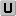Unipartite, undirected Edge typeUnweighted, multiple edges LoopsDoes not contain loops

## Statistics

 Size n = 1,773 Volume m = 16,401 Unique edge count m̿ = 9,131 Loop count l = 0 Wedge count s = 368,148 Claw count z = 17,750,527 Cross count x = 1,124,672,369 Triangle count t = 19,966 Square count q = 321,883 4-Tour count T4 = 4,065,918 Maximum degree dmax = 364 Average degree d = 18.500 8 Fill p = 0.005 812 67 Average edge multiplicity m̃ = 1.796 19 Size of LCC N = 1,707 Diameter δ = 8 50-Percentile effective diameter δ0.5 = 2.839 84 90-Percentile effective diameter δ0.9 = 3.936 54 Median distance δM = 3 Mean distance δm = 3.376 34 Gini coefficient G = 0.688 745 Balanced inequality ratio P = 0.230 169 Relative edge distribution entropy Her = 0.914 733 Power law exponent γ = 1.907 14 Tail power law exponent γt = 2.341 00 Tail power law exponent with p γ3 = 2.341 00 p-value p = 0.000 00 Degree assortativity ρ = −0.048 849 7 Degree assortativity p-value pρ = 3.978 12 × 10−11 Clustering coefficient c = 0.162 701 Spectral norm α = 291.251 Algebraic connectivity a = 0.234 757 Spectral separation |λ1[A] / λ2[A]| = 1.968 42 Non-bipartivity bA = 0.491 978 Normalized non-bipartivity bN = 0.403 984 Algebraic non-bipartivity χ = 0.983 261 Spectral bipartite frustration bK = 0.023 159 6 Controllability C = 43 Relative controllability Cr = 0.024 252 7

## Plots

### Fruchterman–Reingold graph drawing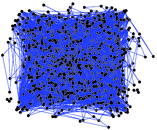### Degree distribution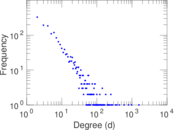### Cumulative degree distribution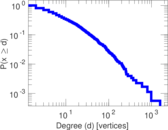### Lorenz curve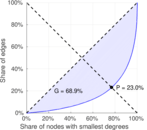### Spectral distribution of the adjacency matrix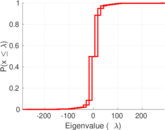### Spectral distribution of the normalized adjacency matrix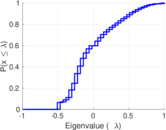### Spectral distribution of the Laplacian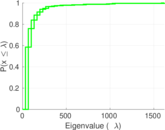### Spectral graph drawing based on the adjacency matrix### Spectral graph drawing based on the Laplacian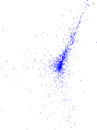### Spectral graph drawing based on the normalized adjacency matrix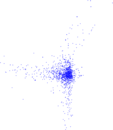### Degree assortativity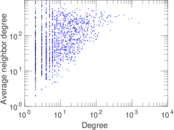### Zipf plot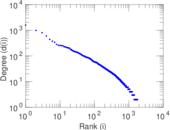### Hop distribution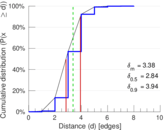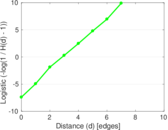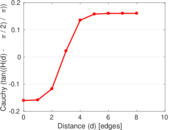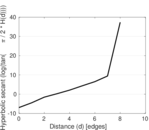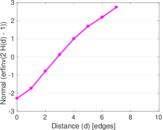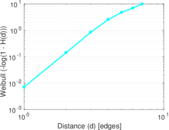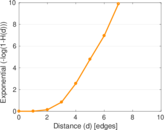### Double Laplacian graph drawing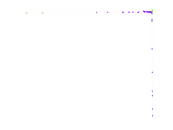### Delaunay graph drawing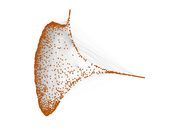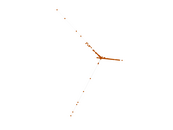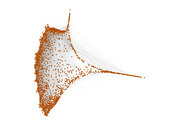### Edge weight/multiplicity distribution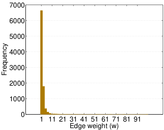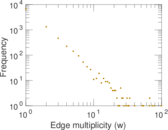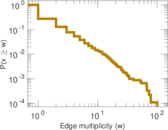### Clustering coefficient distribution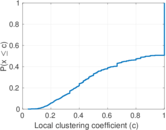### Average neighbor degree distribution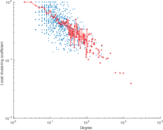### SynGraphy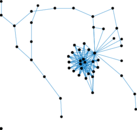### Matrix decompositions plots